# NCERT Solutions for Class 10 Science Chapter 13 Magnetic Effect Of Electric CurrentWritten by Team Trustudies
Updated at 2021-05-07

## NCERT solutions for class 10 Science Chapter 13 Magnetic Effect Of Electric Current Exercise

Q1 )

Which of the following correctly describes the magnetic field near a long straight wire?

1. The field consists of straight lines perpendicular to the wire
2. The field consists of straight lines parallel to the wire
3. The field consists of radial lines originating from the wire
4. The field consists of concentric circles centred on the wire

NCERT Solutions for Class 10 Science Chapter 13 Magnetic Effect Of Electric Current

(d)
The field consists of concentric circles centred on the wire

Q2 )

The phenomenon of electromagnetic induction is

1. the process of charging a body
2. the process of generating magnetic field due to a current passing through a coil
3. producing induced current in a coil due to relative motion between a magnet and the coil
4. the process of rotating a coil of an electric motor

NCERT Solutions for Class 10 Science Chapter 13 Magnetic Effect Of Electric Current

(c)
producing induced current in a coil due to relative motion between a magnet and the coil

Q3 )

The device used for producing electric current is called a

1. generator
2. galvanometer
3. ammeter
4. motor

NCERT Solutions for Class 10 Science Chapter 13 Magnetic Effect Of Electric Current

(a) generator

Q4 )

The essential difference between an AC generator and a DC generator is that

1. AC generator has an electromagnet while a DC generator has permanent magnet.
2. DC generator will generate a higher voltage.
3. AC generator will generate a higher voltage.
4. AC generator has slip rings while the DC generator has a commutator.

NCERT Solutions for Class 10 Science Chapter 13 Magnetic Effect Of Electric Current

(d) AC generator has slip rings while the DC generator has a commutator.

Q5 )

At the time of short circuit, the current in the circuit

1. reduces substantially
2. does not change
3. increases heavily
4. vary continuously

NCERT Solutions for Class 10 Science Chapter 13 Magnetic Effect Of Electric Current

(c) increases heavily

Q6 )

State whether the following statements are true or false.

1. An electric motor converts mechanical energy into electrical energy.
2. (b) An electric generator works on the principle of electromagnetic induction.
3. (c) The field at the centre of a long circular coil carrying current will be parallel straight lines.
4. (d) A wire with a green insulation is usually the live wire of an electric supply.

NCERT Solutions for Class 10 Science Chapter 13 Magnetic Effect Of Electric Current

1. False
An electric motor converts electrical energy into mechanical energy.
2. True
A generator is an electric device that generates electricity by rotating a coil in a magnetic field. It works on the principle of electromagnetic induction.
3. True
The field at the centre of a long circular coil carrying current will be parallel straight lines. A long circular coil is a long solenoid. The magnetic field lines inside the solenoid are parallel.
4. False
A wire with a green insulation is usually the live wire of an electric supply. Live wire has red insulation cover, whereas earth wire has green insulation cover in domestic circuits.

Q7 ) List three sources of magnetic fields.

NCERT Solutions for Class 10 Science Chapter 13 Magnetic Effect Of Electric Current

The sources of magnetic fields are following:-
Electromagnets
Current-carrying conductors
Permanent magnets

Q8 ) How does a solenoid behave like a magnet? Can you determine the north and south poles of a current–carrying solenoid with the help of a bar magnet? Explain.

NCERT Solutions for Class 10 Science Chapter 13 Magnetic Effect Of Electric Current

A solenoid is formed of long coil of circular loops of insulated copper wire.

When a current is allowed to flow through it, magnetic field lines are produced around the solenoid.

The magnetic field produced by it is similar to the magnetic field of a bar magnet.

When the north pole of a bar magnet is brought near the end connected to the negative terminal of the battery, the solenoid repels the bar magnet.

Since like poles repel each other, the end connected to the negative terminal of the battery behaves as the north pole of the solenoid and the other end behaves as a south pole.

Therefore one end of the solenoid behaves as a north pole and the other end behaves as a south pole.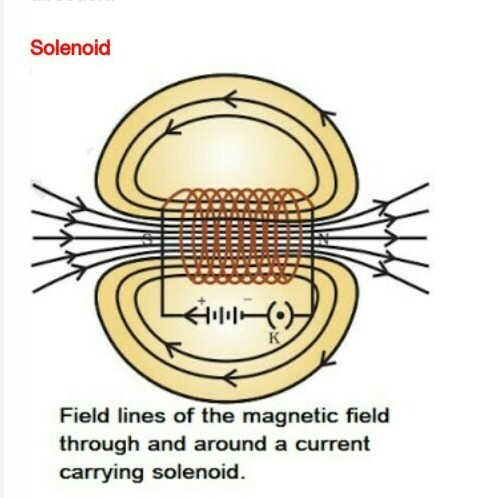Q9 ) When is the force experienced by a current-carrying conductor placed in a magnetic field largest?

NCERT Solutions for Class 10 Science Chapter 13 Magnetic Effect Of Electric Current

The force experienced by a current–carrying conductor placed in a magnetic field largest when the direction of magnetic field and electric current are perpendicular to each other.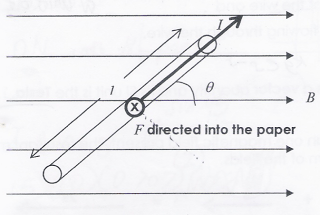Q10 ) Imagine that you are sitting in a chamber with your back to one wall. An electron beam, moving horizontally from back wall towards the front wall, is deflected by a strong magnetic field to your right side. What is the direction of magnetic field?

NCERT Solutions for Class 10 Science Chapter 13 Magnetic Effect Of Electric Current

The direction of the magnetic field is vertically downwards. The direction of current is from the front wall to the back wall because negatively charged electrons are moving from back wall to the front wall. The direction of magnetic force is rightward. Hence, using Fleming's left hand rule, it can be concluded that the direction of magnetic field inside the chamber is downward.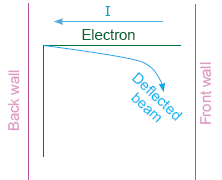Q11 ) Draw a labelled diagram of an electric motor. Explain its principle and working. What is the function of a split ring in an electric motor?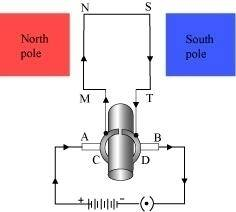NCERT Solutions for Class 10 Science Chapter 13 Magnetic Effect Of Electric Current

Principle: It works on the principle of the magnetic effect of current. A current-carrying coil rotates in a magnetic field.

Working: When a current is allowed to flow through the coil MNST by closing the switch, the coil starts rotating anti-clockwise. This happens because a downward force acts on length MN and at the same time, an upward force acts on length ST. As a result, the coil rotates anti-clockwise.

Current in the length MN flows from M to N and the magnetic field acts from left to right, normal to length MN. Therefore, according to Fleming's left hand rule, a downward force acts on the length MN. Similarly, current in the length ST flows from S to T and the magnetic field acts from left to right, normal to the flow of current. Therefore, an upward force acts on the length ST. These two forces cause the coil to rotate anti-clockwise.

After half a rotation, the position of MN and ST interchange. The half-ring D comes in contact with brush A and half-ring C comes in contact with brush B. Hence, the direction of current in the coil MNST gets reversed.

The current flows through the coil in the direction TSNM. The reversal of current through the coil MNST repeats after each half rotation. As a result, the coil rotates unidirectional.

The split rings help to reverse the direction of current in the circuit. These are called the commutator.

Q12 ) Name some devices in which electric motors are used?

NCERT Solutions for Class 10 Science Chapter 13 Magnetic Effect Of Electric Current

Some devices in which electric motors are Water pumps, Electric fans, Electric mixers and Washing machines.

Q13 ) A coil of insulated copper wire is connected to a galvanometer. What will happen if a bar magnet is
(i) pushed into the coil,
(ii) withdrawn from inside the coil,
(iii) held stationary inside the coil?

NCERT Solutions for Class 10 Science Chapter 13 Magnetic Effect Of Electric Current

(I) When a bar magnet is pushed into the coil then a current is induced momentarily in the coil due to electromagnetic induction . As a result galvanometer shows momentarily deflection.
(II) When a bar magnet is withdrawn from inside the coil, a current is again induced momentarily in the coil but in opposite direction. As a result galvanometer shows momentarily deflection but in opposite direction.
(iii) When a bar magnet is held stationary inside the coil, there will be no current induced in the coil. So there will be no deflection in the galvanometer.

Q14 ) Two circular coils A and B are placed closed to each other. If the current in the coil A is changed, will some current be induced in the coil B? Give reason.

NCERT Solutions for Class 10 Science Chapter 13 Magnetic Effect Of Electric Current

Two circular coils A and B are placed close to each other. When the current in coil A is changed, the magnetic field associated with it also changes. As a result, the magnetic field around coil B also changes. This change in magnetic field lines around coil B induces an electric current in it. This is called electromagnetic induction.Q15 ) State the rule to determine the direction of a (i) magnetic field produced around a straight conductor-carrying current, (ii) force experienced by a current-carrying straight conductor placed in a magnetic field which is perpendicular to it, and (iii) current induced in a coil due to its rotation in a magnetic field.

NCERT Solutions for Class 10 Science Chapter 13 Magnetic Effect Of Electric Current

(i) Maxwell's right hand thumb rule Maxwell’s right hand rule states that if we are holding a current-carrying conductor in our right hand such that the thumb points in the direction of current, then the direction in which fingers encircle, gives the direction of magnetic lines. This rule is also called as Maxwell’s Corkscrew Rule.(ii) Fleming's left hand rule Fleming’s left hand rule states if the forefinger, thumb and middle finger of the left hand are stretched mutually perpendicular and the forefinger points in the direction of external magnetic field; middle finger indicates the direction of current, then the thumb points along the direction of force acting on the conductor.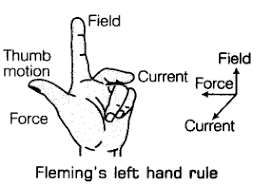(iii) Fleming's right hand rule Fleming’s Right hand rule it states that the states that if we stretch the thumb, forefinger and the middle finger of right hand at right angles to one another in such a way that the forefinger points in the direction of magnetic field; then the thumb gives the direction of motion of conductor (force), forefinger indicates direction of magnetic field and the middle finger points the direction of induced current.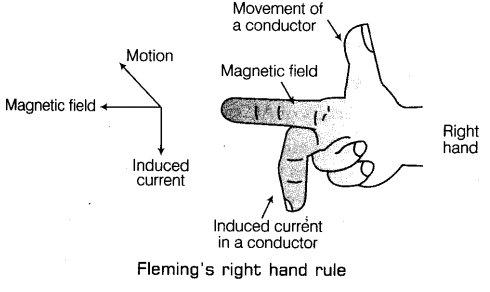Q16 ) Explain the underlying principle and working of an electric generator by drawing a labelled diagram. What is the function of brushes?

NCERT Solutions for Class 10 Science Chapter 13 Magnetic Effect Of Electric Current

Principle: An electric generator works on the principle of electromagnetic induction phenomenon. According to it, whenever a coil is rotated between the poles of a magnet, an induced current is set up in the coil, whose direction is given by Fleming’s right hand rule.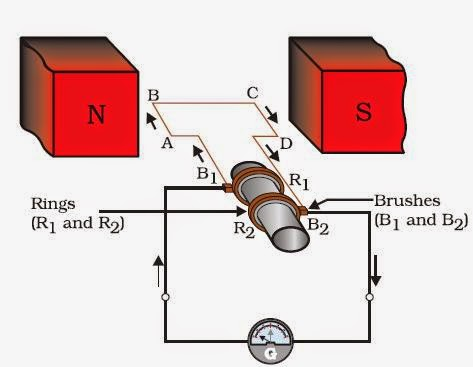Working: Let in the beginning, as shown in Fig. brushes B1 and B2 are kept pressed separately on rings R1 and R2 respectively. Let the axle attached to the rings is rotated such that arm AB of the coil moves up and arm CD moves down in the magnetic field. Due to rotation of arms AB and CD induced currents are set up in them. As per Fleming’s right hand rule induced currents in these arms are along the directions AB and CD. Thus an induced current flows along ABCD and current in the external circuit flows from B2 to B1.

After half a rotation, arm AB starts moving down and the arm CD upward. Therefore, directions of induced currents in these arms change. Thus net induced current now becomes in the direction DCBA. In the external circuit now current flows from B1 to B2. Thus after every half rotation current changes its direction and an alternating current is obtained from the generator.

Function of Brushes: Brushes are kept pressed on the two slip rings separately. Outer ends of the brushes are connected to the galvanometer (or the external load). Thus brushes help in transferring current from the coil ABCD to the external circuit.

Q17 ) When does an electric short circuit occur?

NCERT Solutions for Class 10 Science Chapter 13 Magnetic Effect Of Electric Current

Electric shocks occur when the insulation wire gets damaged, and when the live wire and the neutral wire touch each other, the current flowing in the circuit increases.Also if the resistance of the electric circuit is very low, the current flowing through the circuit increases as a result electric shock can occur.

Q18 ) What is the function of an earth wire? Why is it necessary to earth metallic appliances?

NCERT Solutions for Class 10 Science Chapter 13 Magnetic Effect Of Electric Current

The metallic body of electrical appliance is connected to the earth wire so that any leakage of electric current is transferred to the ground.

This prevents severe shock to the user. That is why earthing of the electrical appliance is necessary.

## NCERT solutions for class 10 Science Chapter 13 Magnetic Effect Of Electric Current Intext Exercise

Q1 ) Why does a compass needle get deflected when brought near a bar magnet?

NCERT Solutions for Class 10 Science Chapter 13 Magnetic Effect Of Electric Current

Compass needle is a small magnet. Thus, when the compass needle and magnet are brought together, the interaction of magnetic fields of the compass needle(small magnet) and the bar magnet leads in the deflection of the compass needle.

Q2 ) Draw magnetic field lines around a bar magnet.

NCERT Solutions for Class 10 Science Chapter 13 Magnetic Effect Of Electric CurrentQ3 ) List the properties of magnetic lines of force.

NCERT Solutions for Class 10 Science Chapter 13 Magnetic Effect Of Electric Current

Properties of magnetic field lines:
(a) Magnetic field lines originate from the north pole and end at the south pole of a magnet.

(b) Magnetic field lines always show concentric patterns but do not cross one another.

(c) Magnetic field lines are closer to each other near the poles; which shows greater strength of magnetic field near the poles but they are widely separated at other places.

Q4 ) Why don’t two magnetic lines of force intersect each other?

NCERT Solutions for Class 10 Science Chapter 13 Magnetic Effect Of Electric Current

If the two field lines cross each other then two tangents can be drawn from that point of intersection which will give two directions of the field from the same point,which is practically impossible.Hence, two magnetic field lines never intersect each other.

Q5 ) Consider a circular loop of wire lying in the plane of the table. Let the current pass through the loop clockwise. Apply the right hand rule to find out the direction of the magnetic field inside and outside the loop.

NCERT Solutions for Class 10 Science Chapter 13 Magnetic Effect Of Electric Current

As the given figure shows; current is flowing clockwise through a circular loop and the direction of the magnetic field around the conductor can be known by using the Right hand thumb rule. As the figure shows,when it is inside the loop, the magnetic field would be towards the plane of the paper and On the other hand, when it is outside the loop, the magnetic field would be away from the paper.Q6 ) The magnetic field in a given region is uniform. Draw a diagram to represent it.

NCERT Solutions for Class 10 Science Chapter 13 Magnetic Effect Of Electric Current

A uniform magnetic field in a region is shown by drawing parallel straight lines, all pointing in the same direction.Q7 ) Choose the correct option.
The magnetic field inside a long straight solenoid-carrying current

(i) is zero

(ii) decreases as we move towards its end

(iii) increases as we move towards its end

(iv) is the same at all points

NCERT Solutions for Class 10 Science Chapter 13 Magnetic Effect Of Electric Current

(iv) Is the same at all points.

Q8 ) Which of the following properties of a proton can change while it moves freely in a magnetic field? (There may be more than one correct answer)
(a) Mass

(b) Speed

(c) Velocity

(d) Momentum

NCERT Solutions for Class 10 Science Chapter 13 Magnetic Effect Of Electric Current

(c) velocity, (d) momentum.

Q9 ) In Activity 13.7, how do we think the displacement of rod AB will be affected if
(i) current in rod AB is increased;
(ii) a stronger horse-shoe magnet is used; and
(iii) length of the rod AB is increased?

NCERT Solutions for Class 10 Science Chapter 13 Magnetic Effect Of Electric Current

(i) When the current in the rod AB is increased, force exerted on the conductor increases, so the displacement of the rod increases.

(ii) When a stronger horse-shoe magnet is used, the magnitude of the magnetic field increases and this increases the force exerted on the rod and the displacement of the rod.

(iii) When the length of the rod AB is increased, force exerted on the conductor increases, so the displacement of the rod increases.

Q10 ) A positively-charged particle (alpha-particle) projected towards west is deflected towards north by a magnetic field. The direction of magnetic field is
(a) Towards south

(b) Towards east

(c) Downward

(d) Upward

NCERT Solutions for Class 10 Science Chapter 13 Magnetic Effect Of Electric Current

(d) Upward.
Here,the direction of current is towards east as the positively charged alpha particles are moving towards west. The deflection is towards north, so the force is towards north, so, we are given that direction of current is towards west and direction of force is towards north.

Let us now hold the forefinger, middle finger and thumb of our left-hand at right angles to one another as per Fleming’s left hand rule. Adjust the hand in such a way that our mid finger points towards west (in the direction of current) and thumb points towards north (in the direction of force). Now, if we look at our forefinger, it will be pointing upward.So the magnetic field is in the upward direction because the direction of the forefinger gives the direction of the magnetic field.

Q11 ) State Fleming’s left hand rule.

NCERT Solutions for Class 10 Science Chapter 13 Magnetic Effect Of Electric Current

Fleming’s left hand rule states that when a current-carrying conductor is placed in an external magnetic field, the conductor experiences a force perpendicular to both the field and to the direction of the current flow. And the direction of the different parameters can be identified as:Stretch the first finger, the middle finger and the thumb of your left hand mutually perpendicular to each other in such a way that the first finger represents the direction of the magnetic field, the middle finger represents the direction of the current in the conductor, then the thumb will represent the direction of motion of the conductor.

Q12 ) What is the principle of an electric motor ?

NCERT Solutions for Class 10 Science Chapter 13 Magnetic Effect Of Electric Current

The electric motor works on the principle of Fleming’s Left Hand Rule which states that when a rectangular coil is placed within a magnetic field and current is passed through the coil, a force acts on the coil which rotates it continuously. When the coil rotates, the shaft attached to it also rotates and in this way the electrical energy supplied to the motor is converted into the mechanical energy of rotation.

Q13 ) What is the role of the split ring in an electric motor ?

NCERT Solutions for Class 10 Science Chapter 13 Magnetic Effect Of Electric Current

The split ring reverses the direction of current in the armature coil after every half rotation due to change in orientation of the magnetic field, i.e, it acts as a commutator. The reversed current reverses the direction of the forces acting on the two arms of the armature after every half rotation and this allows the armature coil to rotate continuously in the same direction.

Q14 ) Explain different ways to induce current in a coil.

NCERT Solutions for Class 10 Science Chapter 13 Magnetic Effect Of Electric Current

Different ways to induce current in a coil are :
(i)Inducing current on a coil by moving the coil in a magnetic field or inducing current on a coil by changing the magnetic field across it.

(ii)By taking two conducting coils and inserting them and then on changing current flowing in one coil, an induced current is obtained in the other coil.

Q15 ) State the principle of an electric generator.

NCERT Solutions for Class 10 Science Chapter 13 Magnetic Effect Of Electric Current

The electric generator works on the principle of electromagnetic induction which obeys Fleming’s Right Hand Rule i.e, when a conductor is moved in a magnetic field, then a current is induced in the conductor.Thus in this way, the electric current is thus ‘generated’ in the electric generator.

Q16 ) Name some sources of direct current.

NCERT Solutions for Class 10 Science Chapter 13 Magnetic Effect Of Electric Current

Some of the sources of direct current are dry cells, lead accumulators, DC generator,etc.

Q17 ) Which sources produce alternating current ?

NCERT Solutions for Class 10 Science Chapter 13 Magnetic Effect Of Electric Current

Alternating current is produced by AC generators .

Q18 ) Choose the correct option : A rectangular coil of copper wires is rotated in a magnetic field. The direction of the induced current changes once in each:
(i) two revolution

(ii) one revolution

(iii) half revolution

(iv) one-fourth revolution

NCERT Solutions for Class 10 Science Chapter 13 Magnetic Effect Of Electric Current

(iii) Half revolution.

Q19 ) Name two safety measures commonly used in electric circuits and appliances.

NCERT Solutions for Class 10 Science Chapter 13 Magnetic Effect Of Electric Current

(i) Earthing(earth wire)
(ii) Electric fuse.

Q20 ) An electric oven of 2 kW power rating is operated in a domestic electric circuit (220 V) that has a current rating of 5 A. What result do you expect ? Explain.

NCERT Solutions for Class 10 Science Chapter 13 Magnetic Effect Of Electric Current

We have formula as P=VI
The electric oven draws a current given by

$$I=\frac{P}V=\frac{2kW}{220V}=\frac{2000}{220}=9.09A$$

Thus the electric oven draws much more than the current rating 5 A. thus, the circuit is overloaded so the fuse wire will blow and the circuit will break.

Q21 ) What precautions should be taken to avoid the overloading of domestic electric circuits ?

NCERT Solutions for Class 10 Science Chapter 13 Magnetic Effect Of Electric Current

To avoid the overloading of domestic electric circuits, the following precautions should be taken :

(i) Coating on the wires used in the circuit must be with good insulating materials like PVC, etc.

(ii) The circuit must be divided into different sections and a safety fuse or MCB must be used in each section.

(iii) High power appliances or too many appliances like air-conditioner, refrigerator, a water heater, etc. should not be used in a single line, i.e. from a single output.

##### FAQs Related to NCERT Solutions for Class 10 Science Chapter 13 Magnetic Effect Of Electric Current
There are total 39 questions present in ncert solutions for class 10 science chapter 13 magnetic effect of electric current
There are total 3 long question/answers in ncert solutions for class 10 science chapter 13 magnetic effect of electric current
There are total 2 exercise present in ncert solutions for class 10 science chapter 13 magnetic effect of electric current